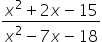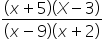# CAT-2021-QA-Slot-1/Free CAT Question papers

4).

f(x) =is negative if and only if

1. -5 < x < -2 or 3 < x < 9

2. -2 < x < 3 or x > 9

3. x < -5 or 3 < x < 9

4. x < -5 or -2 < x < 3

### Explanation

By taking factors of numerator and denominator, the function can be written as

f(x) =The function will be negative if and only if one or three of the factors are negative.

When x < -5, all factors are negative. If -5 < x < - 2, only (x +5) is positive. If  3 < x < 9, only (x – 9) is negative.

Thus, f(x) =is negative if and only if  -5 < x < -2 or  3 < x < 9

Ans (1)